Why do I hate zeros in my dataset?9 months agoIt is always good practice to explore the data before you fit a model. A clear understanding of the dataset helps you to select the appropriate statistical approach and, in the case of linear models, to identify the corresponding design and treatment structure by defining relevant variates and factors.

So, I have in my hands a dataset from a given study, and I proceed to explore it, maybe to do some data cleaning, but mainly to get familiar with it. Assessing predictors is important but more critical is to evaluate the single or multiple response variables that need to be analysed. And it is in these columns where I often find surprises. Sometimes they contain not only numbers, as they should for linear model responses, but also non-numeric data. I have found comments (‘missing’ or ‘not found’), letters (‘?’), and one or more definitions of missing values (‘NA’, ‘NaN’, ‘*’, ‘.’ or even ‘-9’). But what is the most disturbing to me is the ZEROS, and I especially hate them when they come in masses!

But why do zeros make me angry?! Because their definition is not clear, and they can be the cause of large errors, and ultimately incorrect models. Here are some of my reasons…

Missing Values

First, it is common to use zero as the definition for missing values. For example, a plant that did not have any fruit has a zero value. But what if the plant died before fruiting? Yes, it will have zero fruits, but here the experimental unit (the plant) no longer exists. In this case, there is a big difference between a true zero that was observed and a zero because of missing data.

Default Values

Second, zeros are sometimes used as default values in the columns of spreadsheets. That is, you start with a column of zeros that is replaced by true records. However, for many reasons data points may not be collected (for example, you could skip measuring your last replication), and hence some cells of the spreadsheet are not visited, and their values are unchanged from the zero default. Again, these are true missing values, and therefore they need to be recorded in a way that indicates that they were not observed!

Third, zeros are often values reflecting measurements that are below the detection limit. For example, if the weighing balance precision is <0.5 grams then any weight of seed below 0.5 grams will be recorded as a zero. Yes, we do have a range of seed weights reaching 23 grams, and a small portion might be below 1 gram, but in this case the zeros are not really zeros, they approximate a true unknown record between 0 and 0.5 grams.

When, under an initial exploration of the dataset we discover that there are lots of zeros, we need to question why they are occurring. Of course, conversations with the researcher and the staff doing the data recording will give critical insight. This should help us identify the true zeros from the false ones. If there are no missing values recorded in the data, then we might think that some of these zeros are missing values. Here is where I like to explore additional columns (e.g., survival notes) to help ‘recover’ the missing values. However, it might be impossible to discriminate between the true zeros and the missing values if this extra information was not recorded in the dataset. This unfortunate situation, to the misfortune of my collaborators, might mean that the dataset must be completely discarded.

In the case of missing values due to detection limits, the best approach is to ask the researcher. Here, I like to first make sure that this is really the case, and from there make an educated decision on how to analyse the data. Replacing undetected observations by a zero creates two undesired issues:

1. A bias, as these values are not zero, but for example, as in our previous case they have an average value of 0.25 grams (i.e., half the detection limit), and
2. Reduced background variability, as all undetected observations are recorded with exactly the same value when in fact they are not identical, but we can’t see this variability!

Finally, there is another reason for me to hate zeros. Suppose that they are all verified valid numbers, but that we still have a high proportion of zeros in our dataset. For example, in a study on fruit yield, I might have 20% of live plants producing no fruit, resulting in 20% true zeros in my dataset. This large proportion of zeros creates difficulties for traditional statistical analyses. For example, when fitting a linear model, the assumption of an approximate Normal distribution might no longer hold, and this will be reflected in residual plots with a strange appearance!

So, what is the solution for this ‘excess’ of zeros? In some cases, a simple transformation could reduce the influence of these zeros in my analyses. Often, the most logical alternative is to rethink the biological process to model, and this might require something different than our typical statistical tools. For example, we could separate the process into two parts. The first part separates the zeros from the non-zeros using a Binomial model that includes several explanatory variables (e.g., age, size, sex). The second part deals only with the non-zero values and fits another model based on, say a Normal distribution, that will include the same or other explanatory variables, but in this case we model the magnitude of this response. This is the basis of some of the Hurdle models, but other statistical approaches, particularly Bayesian, are also available.

In summary, I have many reasons to hate zeros, and you might have a few additional ones. However, I believe they are a critical part of data exploration: not only they can be the tip of an iceberg leading to a better understanding and modelling of the process under which the data was obtained, but they also help to identify potentially more adequate models to describe the system. Hence, perhaps I should embrace the zeros in my dataset and not be so angry about them!

April, 2021Kanchana Punyawaew

a year ago

Linear mixed models: a balanced lattice square

This blog illustrates how to analyze data from a field experiment with a balanced lattice square design using linear mixed models. We’ll consider two models: the balanced lattice square model and a spatial model.

The example data are from a field experiment conducted at Slate Hall Farm, UK, in 1976 (Gilmour et al., 1995). The experiment was set up to compare the performance of 25 varieties of barley and was designed as a balanced lattice square with six replicates laid out in a 10 x 15 rectangular grid. Each replicate contained exactly one plot for every variety. The variety grown in each plot, and the coding of the replicates and lattice blocks, is shown in the field layout below: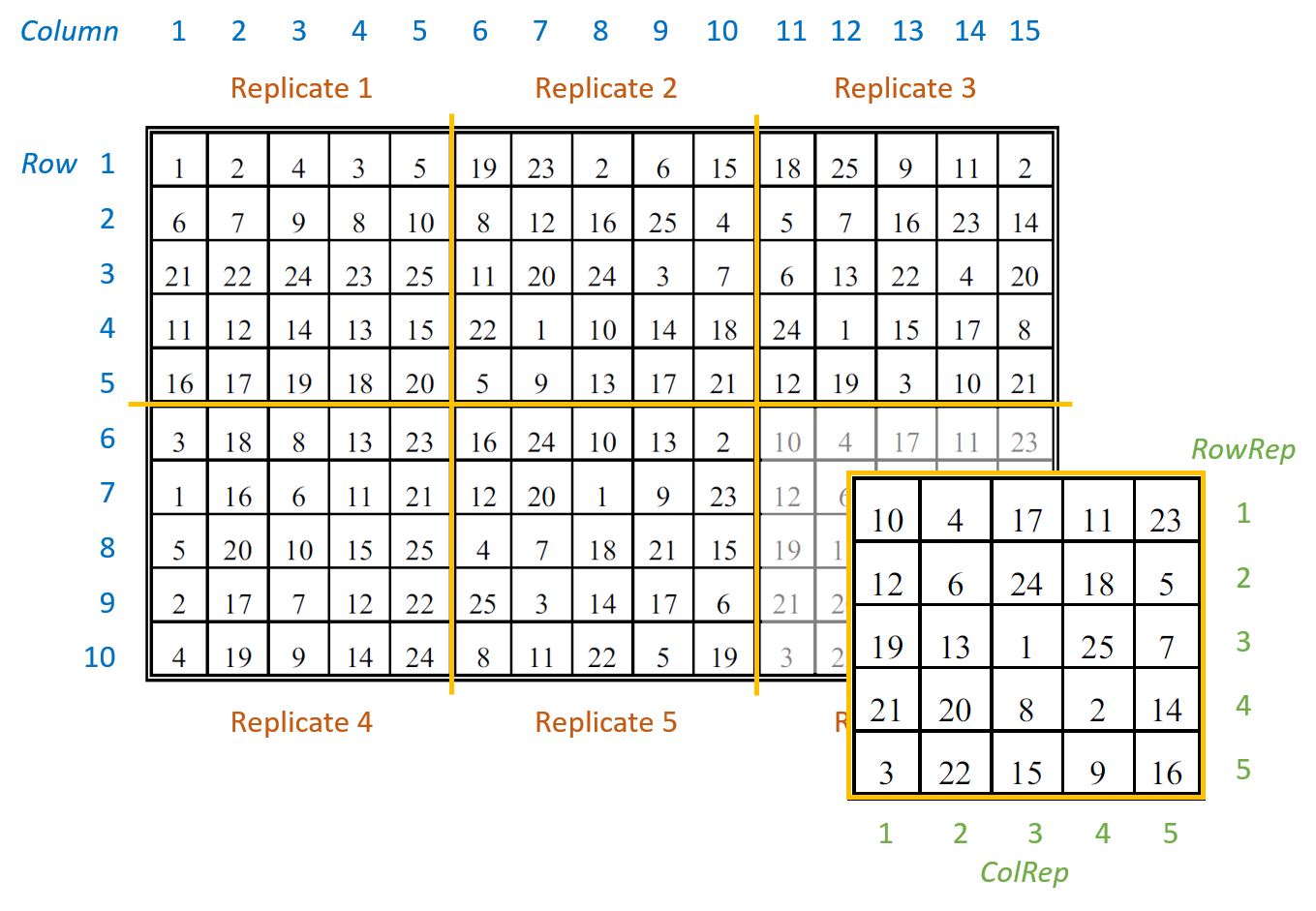There are seven columns in the data frame: five blocking factors (Rep, RowRep, ColRep, Row, Column), one treatment factor, Variety, and the response variate, yield.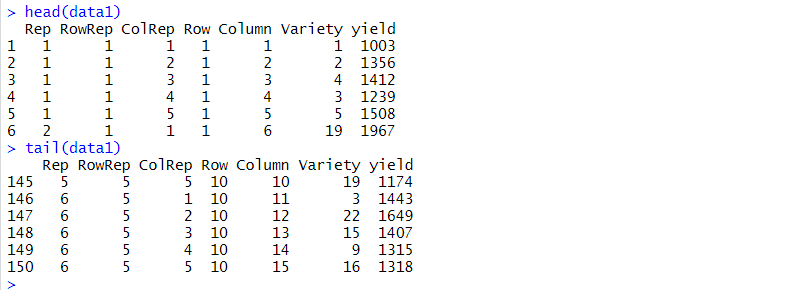The six replicates are numbered from 1 to 6 (Rep). The lattice block numbering is coded within replicates. That is, within each replicates the lattice rows (RowRep) and lattice columns (ColRep) are both numbered from 1 to 5. The Row and Column factors define the row and column positions within the field (rather than within each replicate).

Analysis of a balanced lattice square design

To analyze the response variable, yield, we need to identify the two basic components of the experiment: the treatment structure and the blocking (or design) structure. The treatment structure consists of the set of treatments, or treatment combinations, selected to study or to compare. In our example, there is one treatment factor with 25 levels, Variety (i.e. the 25 different varieties of barley). The blocking structure of replicates (Rep), lattice rows within replicates (Rep:RowRep), and lattice columns within replicates (Rep:ColRep) reflects the balanced lattice square design. In a mixed model analysis, the treatment factors are (usually) fitted as fixed effects and the blocking factors as random.

The balanced lattice square model is fitted in ASReml-R4 using the following code:

> lattice.asr <- asreml(fixed = yield ~ Variety,
random = ~ Rep + Rep:RowRep + Rep:ColRep,
data=data1)

The REML log-likelihood is -707.786.

The model’s BIC is: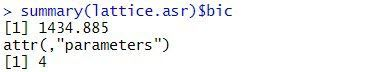The estimated variance components are: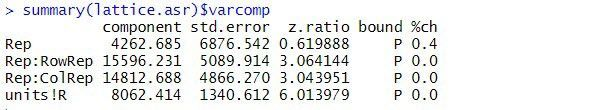The table above contains the estimated variance components for all terms in the random model. The variance component measures the inherent variability of the term, over and above the variability of the sub-units of which it is composed. The variance components for Rep, Rep:RowRep and Rep:ColRep are estimated as 4263, 15596, and 14813, respectively. As is typical, the largest unit (replicate) is more variable than its sub-units (lattice rows and columns within replicates). The "units!R" component is the residual variance.

By default, fixed effects in ASReml-R4 are tested using sequential Wald tests: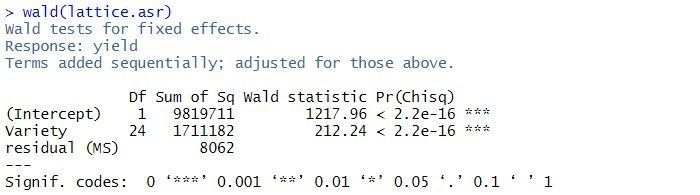In this example, there are two terms in the summary table: the overall mean, (Intercept), and Variety. As the tests are sequential, the effect of the Variety is assessed by calculating the change in sums of squares between the two models (Intercept)+Variety and (Intercept). The p-value (Pr(Chisq)) of  < 2.2 x 10-16 indicates that Variety is a highly significant.

The predicted means for the Variety can be obtained using the predict() function. The standard error of the difference between any pair of variety means is 62. Note: all variety means have the same standard error as the design is balanced.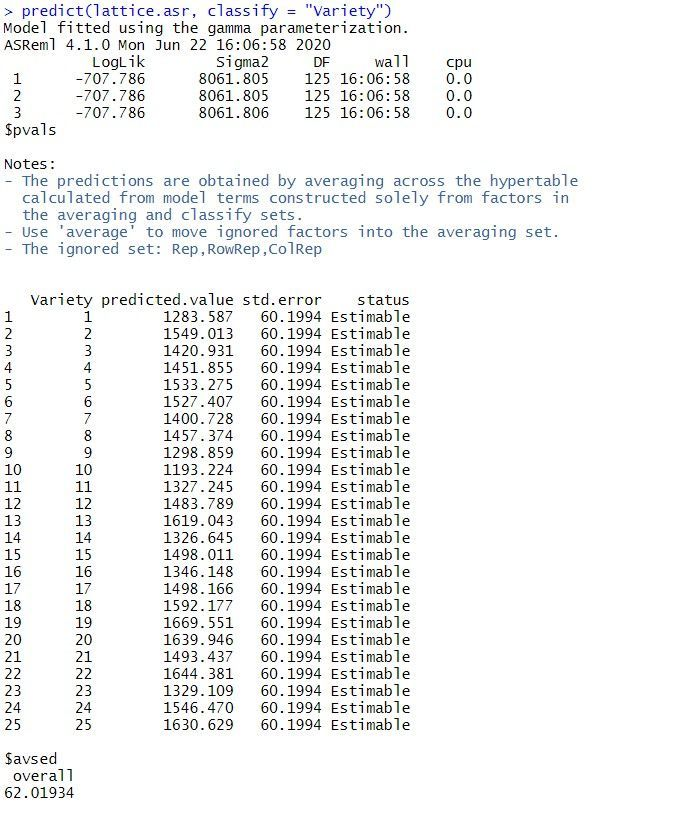Note: the same analysis is obtained when the random model is redefined as replicates (Rep), rows within replicates (Rep:Row) and columns within replicates (Rep:Column).

Spatial analysis of a field experiment

As the plots are laid out in a grid, the data can also be analyzed using a spatial model. We’ll illustrate spatial analysis by fitting a model with a separable first order autoregressive process in the field row (Row) and field column (Column) directions. This is often a useful model to start the spatial modeling process.

The separable first order autoregressive spatial model is fitted in ASReml-R4 using the following code:

> spatial.asr <- asreml(fixed = yield ~ Variety,
residual = ~ar1(Row):ar1(Column),
data = data1)

The BIC for this spatial model is: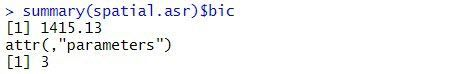The estimated variance components and sequential Wald tests are: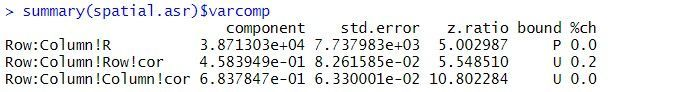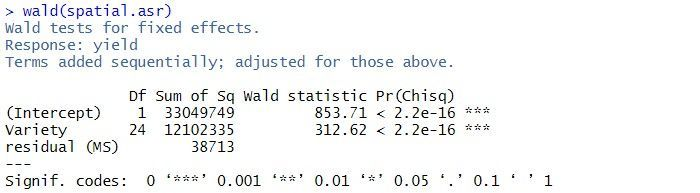The residual variance is 38713, the estimated row correlation is 0.458, and the estimated column correlation is 0.684. As for the balanced lattice square model, there is strong evidence of a Variety effect (p-value < 2.2 x 10-16).

A log-likelihood ratio test cannot be used to compare the balanced lattice square model with the spatial models, as the variance models are not nested. However, the two models can be compared using BIC. As the spatial model has a smaller BIC (1415) than the balanced lattice square model (1435), of the two models explored in this blog, it is chosen as the preferred model. However, selecting the optimal spatial model can be difficult. The current spatial model can be extended by including measurement error (or nugget effect) or revised by selecting a different variance model for the spatial effects.

References

Butler, D.G., Cullis, B.R., Gilmour, A. R., Gogel, B.G. and Thompson, R. (2017). ASReml-R Reference Manual Version 4. VSN International Ltd, Hemel Hempstead, HP2 4TP UK.

Gilmour, A. R., Anderson, R. D. and Rae, A. L. (1995). The analysis of binomial data by a generalised linear mixed model, Biometrika 72: 593-599..Kanchana Punyawaew and Dr. Vanessa Cave

a year ago

Mixed models for repeated measures and longitudinal data

The term "repeated measures" refers to experimental designs or observational studies in which each experimental unit (or subject) is measured repeatedly over time or space. "Longitudinal data" is a special case of repeated measures in which variables are measured over time (often for a comparatively long period of time) and duration itself is typically a variable of interest.

In terms of data analysis, it doesn’t really matter what type of data you have, as you can analyze both using mixed models. Remember, the key feature of both types of data is that the response variable is measured more than once on each experimental unit, and these repeated measurements are likely to be correlated.

Mixed Model Approaches

To illustrate the use of mixed model approaches for analyzing repeated measures, we’ll examine a data set from Landau and Everitt’s 2004 book, “A Handbook of Statistical Analyses using SPSS”. Here, a double-blind, placebo-controlled clinical trial was conducted to determine whether an estrogen treatment reduces post-natal depression. Sixty three subjects were randomly assigned to one of two treatment groups: placebo (27 subjects) and estrogen treatment (36 subjects). Depression scores were measured on each subject at baseline, i.e. before randomization (predep) and at six two-monthly visits after randomization (postdep at visits 1-6). However, not all the women in the trial had their depression score recorded on all scheduled visits.

In this example, the data were measured at fixed, equally spaced, time points. (Visit is time as a factor and nVisit is time as a continuous variable.) There is one between-subject factor (Group, i.e. the treatment group, either placebo or estrogen treatment), one within-subject factor (Visit or nVisit) and a covariate (predep).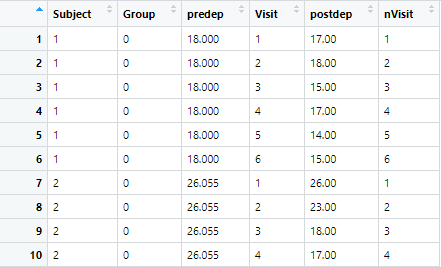Using the following plots, we can explore the data. In the first plot below, the depression scores for each subject are plotted against time, including the baseline, separately for each treatment group.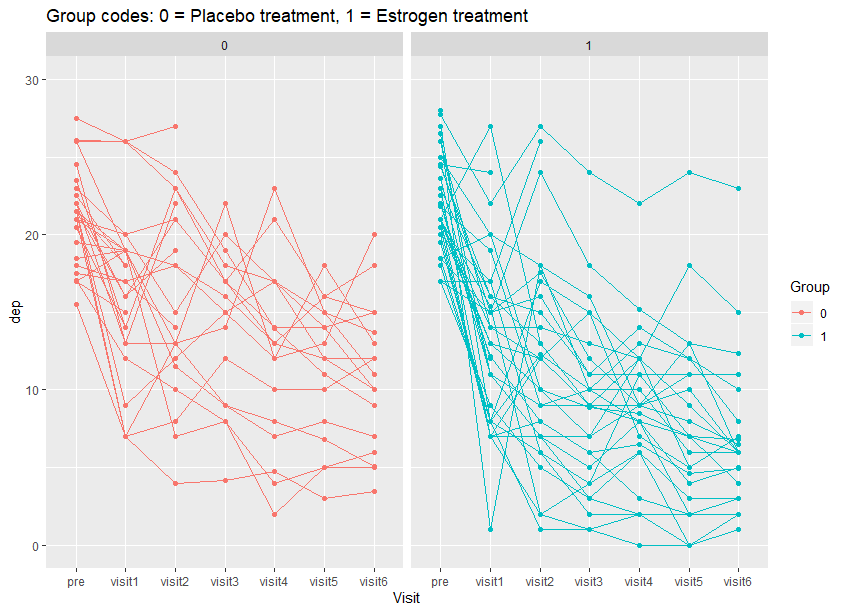In the second plot, the mean depression score for each treatment group is plotted over time. From these plots, we can see variation among subjects within each treatment group that depression scores for subjects generally decrease with time, and on average the depression score at each visit is lower with the estrogen treatment than the placebo.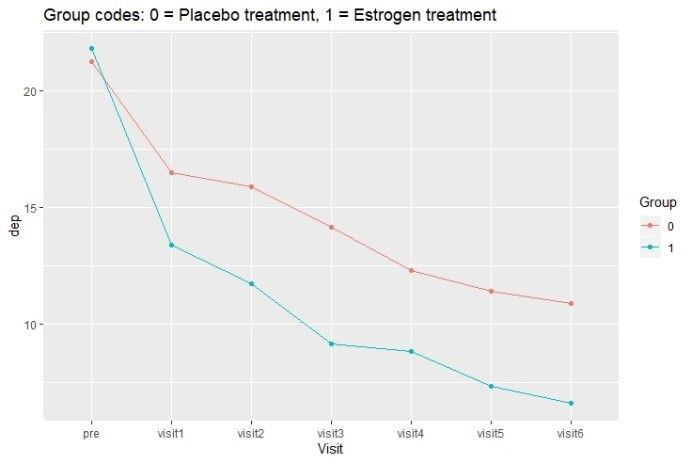Random effects model

The simplest approach for analyzing repeated measures data is to use a random effects model with subject fitted as random. It assumes a constant correlation between all observations on the same subject. The analysis objectives can either be to measure the average treatment effect over time or to assess treatment effects at each time point and to test whether treatment interacts with time.

In this example, the treatment (Group), time (Visit), treatment by time interaction (Group:Visit) and baseline (predep) effects can all be fitted as fixed. The subject effects are fitted as random, allowing for constant correlation between depression scores taken on the same subject over time.

The code and output from fitting this model in ASReml-R 4 follows;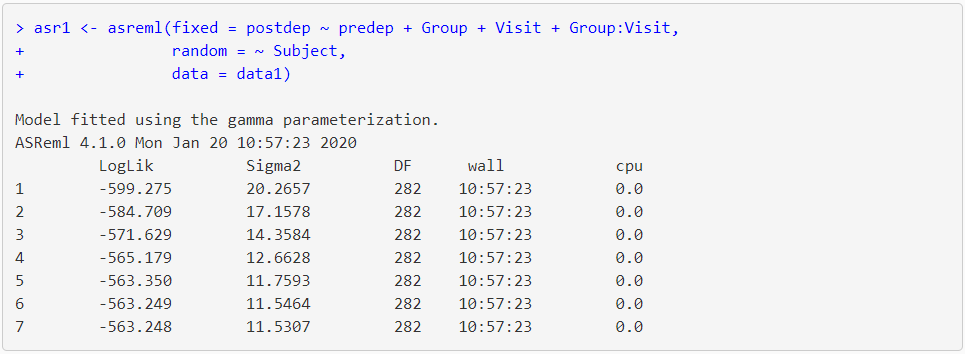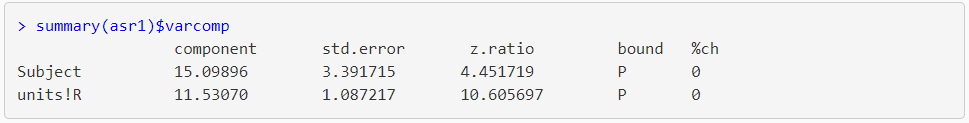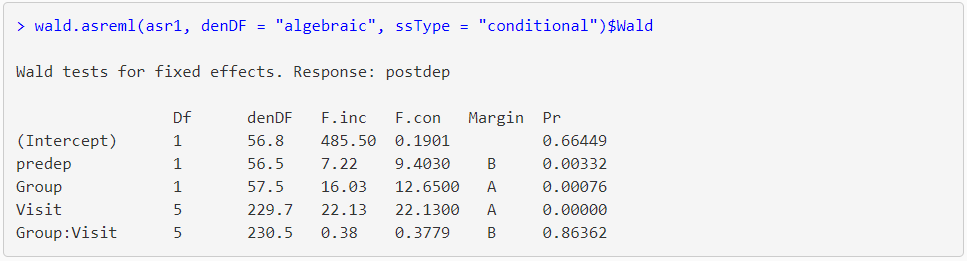The output from summary() shows that the estimate of subject and residual variance from the model are 15.10 and 11.53, respectively, giving a total variance of 15.10 + 11.53 = 26.63. The Wald test (from the wald.asreml() table) for predep, Group and Visit are significant (probability level (Pr) ≤ 0.01). There appears to be no relationship between treatment group and time (Group:Visit) i.e. the probability level is greater than 0.05 (Pr = 0.8636).

Covariance model

In practice, often the correlation between observations on the same subject is not constant. It is common to expect that the covariances of measurements made closer together in time are more similar than those at more distant times. Mixed models can accommodate many different covariance patterns. The ideal usage is to select the pattern that best reflects the true covariance structure of the data. A typical strategy is to start with a simple pattern, such as compound symmetry or first-order autoregressive, and test if a more complex pattern leads to a significant improvement in the likelihood.

Note: using a covariance model with a simple correlation structure (i.e. uniform) will provide the same results as fitting a random effects model with random subject.

In ASReml-R 4 we use the corv() function on time (i.e. Visit) to specify uniform correlation between depression scores taken on the same subject over time.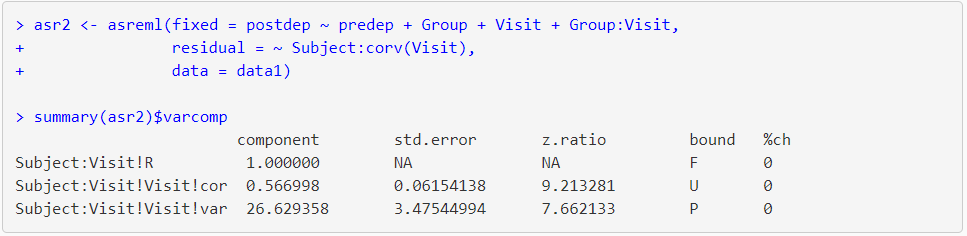Here, the estimate of the correlation among times (Visit) is 0.57 and the estimate of the residual variance is 26.63 (identical to the total variance of the random effects model, asr1).

Specifying a heterogeneous first-order autoregressive covariance structure is easily done in ASReml-R 4 by changing the variance-covariance function in the residual term from corv() to ar1h().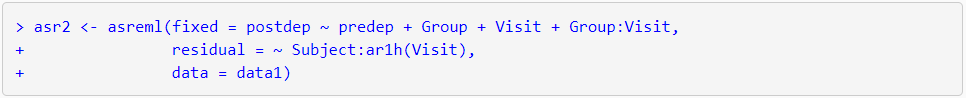Random coefficients model

When the relationship of a measurement with time is of interest, a random coefficients model is often appropriate. In a random coefficients model, time is considered a continuous variable, and the subject and subject by time interaction (Subject:nVisit) are fitted as random effects. This allows the slopes and intercepts to vary randomly between subjects, resulting in a separate regression line to be fitted for each subject. However, importantly, the slopes and intercepts are correlated.

The str() function of asreml() call is used for fitting a random coefficient model;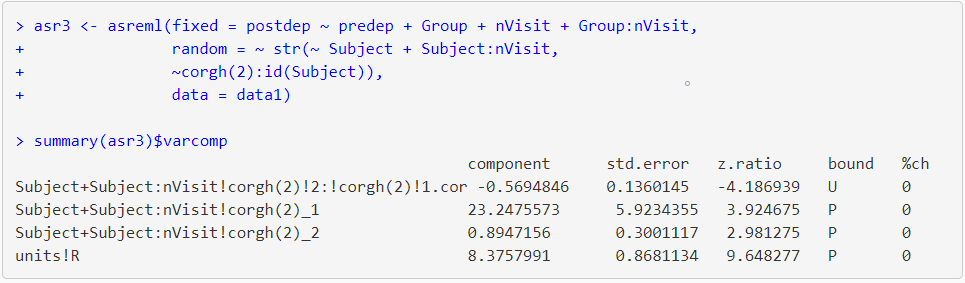The summary table contains the variance parameter for Subject (the set of intercepts, 23.24) and Subject:nVisit (the set of slopes, 0.89), the estimate of correlation between the slopes and intercepts (-0.57) and the estimate of residual variance (8.38).

References

Brady T. West, Kathleen B. Welch and Andrzej T. Galecki (2007). Linear Mixed Models: A Practical Guide Using Statistical Software. Chapman & Hall/CRC, Taylor & Francis Group, LLC.

Brown, H. and R. Prescott (2015). Applied Mixed Models in Medicine. Third Edition. John Wiley & Sons Ltd, England.

Sabine Landau and Brian S. Everitt (2004). A Handbook of Statistical Analyses using SPSS. Chapman & Hall/CRC Press LLC.The VSNi Team

9 months ago

Evolution of statistical computing

It is widely acknowledged that the most fundamental developments in statistics in the past 60+ years are driven by information technology (IT). We should not underestimate the importance of pen and paper as a form of IT but it is since people start using computers to do statistical analysis that we really changed the role statistics plays in our research as well as normal life.

In this blog we will give a brief historical overview, presenting some of the main general statistics software packages developed from 1957 onwards. Statistical software developed for special purposes will be ignored. We also ignore the most widely used ‘software for statistics’ as Brian Ripley (2002) stated in his famous quote: “Let’s not kid ourselves: the most widely used piece of software for statistics is Excel.” Our focus is some of the packages developed by statisticians for statisticians, which are still evolving to incorporate the latest development of statistics.

Ronald Fisher’s Calculating Machines

Pioneer statisticians like Ronald Fisher started out doing their statistics on pieces of paper and later upgraded to using calculating machines. Fisher bought the first Millionaire calculating machine when he was heading Rothamsted Research’s statistics department in the early 1920s. It cost about £200 at that time, which is equivalent in purchasing power to about £9,141 in 2020. This mechanical calculator could only calculate direct product, but it was very helpful for the statisticians at that time as Fisher mentioned: "Most of my statistics has been learned on the machine." The calculator was heavily used by Fisher’s successor Frank Yates (Head of Department 1933-1968) and contributed to much of Yates’ research, such as designs with confounding between treatment interactions and blocks, or split plots, or quasi-factorials.Frank Yates

Rothamsted Annual Report for 1952: "The analytical work has again involved a very considerable computing effort."

Beginning of the Computer Age

From the early 1950s we entered the computer age. The computer at this time looked little like its modern counterpart, whether it was an Elliott 401 from the UK or an IBM 700/7000 series in the US. Although the first documented statistical package, BMDP, was developed starting in 1957 for IBM mainframes at the UCLA Health Computing Facility, on the other side of the Atlantic Ocean statisticians at Rothamsted Research began their endeavours to program on an Elliot 401 in 1954.Programming Statistical Software

When we teach statistics in schools or universities, students very often complain about the difficulties of programming. Looking back at programming in the 1950s will give modern students an appreciation of how easy programming today actually is!

An Elliott 401 served one user at a time and requested all input on paper tape (forget your keyboard and intelligent IDE editor). It provided the output to an electric typewriter. All programming had to be in machine code with the instructions and data on a rotating disk with 32-bit word length, 5 "words" of fast-access store, 7 intermediate access tracks of 128 words, 16 further tracks selectable one at a time (= 2949 words – 128 for system).Computer paper tape

fitting constants to main effects and interactions in multi-way tables (1957), regression and multiple regression (1956), fitting many standard curves as well as multivariate analysis for latent roots and vectors (1955).

Although it sounds very promising with the emerging of statistical programs for research, routine statistical analyses were also performed and these still represented a big challenge, at least computationally. For example, in 1963, which was the last year with the Elliott 401 and Elliott 402 computers, Rothamsted Research statisticians analysed 14,357 data variables, and this took them 4,731 hours to complete the job. It is hard to imagine the energy consumption as well as the amount of paper tape used for programming. Probably the paper tape (all glued together) would be long enough to circle the equator.

Development of Statistical Software: Genstat, SAS, SPSS

The above collection of programs was mainly used for agricultural research at Rothamsted and was not given an umbrella name until John Nelder became Head of the Statistics Department in 1968. The development of Genstat (General Statistics) started from that year and the programming was done in FORTRAN, initially on an IBM machine. In that same year, at North Carolina State University, SAS (Statistical Analysis Software) was almost simultaneously developed by computational statisticians, also for analysing agricultural data to improve crop yields. At around the same time, social scientists at the University of Chicago started to develop SPSS (Statistical Package for the Social Sciences). Although the three packages (Genstat, SAS and SPSS) were developed for different purposes and their functions diverged somewhat later, the basic functions covered similar statistical methodologies.

The first version of SPSS was released in 1968. In 1970, the first version of Genstat was released with the functions of ANOVA, regression, principal components and principal coordinate analysis, single-linkage cluster analysis and general calculations on vectors, matrices and tables. The first version of SAS, SAS 71, was released and named after the year of its release. The early versions of all three software packages were written in FORTRAN and designed for mainframe computers.

Since the 1980s, with the breakthrough of personal computers, a second generation of statistical software began to emerge. There was an MS-DOS version of Genstat (Genstat 4.03) released with an interactive command line interface in 1980.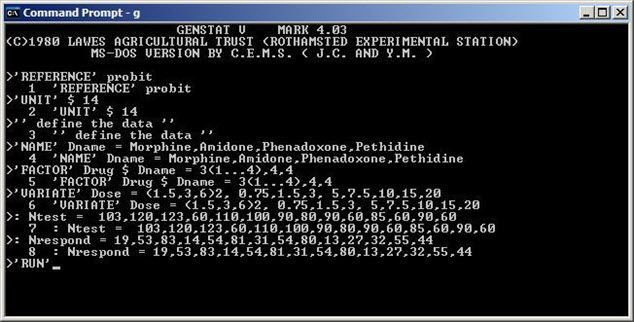Genstat 4.03 for MSDOS

Around 1985, SAS and SPSS also released a version for personal computers. In the 1980s more players entered this market: STATA was developed from 1985 and JMP was developed from 1989. JMP was, from the very beginning, for Macintosh computers. As a consequence, JMP had a strong focus on visualization as well as graphics from its inception.

The Rise of the Statistical Language R

The development of the third generation of statistical computing systems had started before the emergence of software like Genstat 4.03e or SAS 6.01. This development was led by John Chambers and his group in Bell Laboratories since the 1970s. The outcome of their work is the S language. It had been developed into a general purpose language with implementations for classical as well as modern statistical inferences. S language was freely available, and its audience was mainly sophisticated academic users. After the acquisition of S language by the Insightful Corporation and rebranding as S-PLUS, this leading third generation statistical software package was widely used in both theoretical and practical statistics in the 1990s, especially before the release of a stable beta version of the free and open-source software R in the year 2000. R was developed by Ross Ihaka and Robert Gentleman at the University of Auckland, New Zealand, and is currently widely used by statisticians in academia and industry, together with statistical software developers, data miners and data analysts.

Software like Genstat, SAS, SPSS and many other packages had to deal with the challenge from R. Each of these long-standing software packages developed an R interface R or even R interpreters to anticipate the change of user behaviour and ever-increasing adoption of the R computing environment. For example, SAS and SPSS have some R plug-ins to talk to each other. VSNi’s ASReml-R software was developed for ASReml users who want to run mixed model analysis within the R environment, and at the present time there are more ASReml-R users than ASReml standalone users. Users who need reliable and robust mixed effects model fitting adopted ASReml-R as an alternative to other mixed model R packages due to its superior performance and simplified syntax. For Genstat users, msanova was also developed as an R package to provide traditional ANOVA users an R interface to run their analysis.

What’s Next?

We have no clear idea about what will represent the fourth generation of statistical software. R, as an open-source software and a platform for prototyping and teaching has the potential to help this change in statistical innovation. An example is the R Shiny package, where web applications can be easily developed to provide statistical computing as online services. But all open-source and commercial software has to face the same challenges of providing fast, reliable and robust statistical analyses that allow for reproducibility of research and, most importantly, use sound and correct statistical inference and theory, something that Ronald Fisher will have expected from his computing machine!